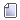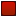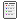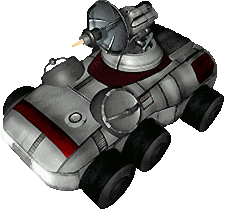###Author Topic: Delegates, std::function, and std::bind  (Read 4781 times)

####Hooman

• Hero Member
••• Posts: 4952##### Delegates, std::function, and std::bind
« on: October 12, 2018, 02:28:39 PM »
I did a bit of reading around the topic of delegates after a brief discussion about signals and slots with Leeor.

Bjarne Stroustrup had a list of C++11 features, which included a section on std::function. Of particular note is the section towards the end, it states a member function is treated the same as a free function with an extra argument (corresponding to this). He called the function both explicitly passing this, and using std::bind to fix the this pointer to a constant value, making it callable the same way as a free function:
Code: cpp [Select]
``struct X {  int foo(int);};function<int (X*, int)> f;f = &X::foo;		// pointer to memberX x;int v = f(&x, 5);	// call X::foo() for x with 5function<int (int)> ff = std::bind(f,&x,_1);	// first argument for f is &xv=ff(5); 		// call x.foo(5)``

Here's some reference info:
std::function
std::bind

The std::function type is general-purpose polymorphic function wrapper. It holds arbitrary references to callable types. What matters is the signature of the call, not the type of the underlying Callable target. It can be assigned from a free function, a member function, a member data variable, a functor (an object which overloads operator()), and lambdas (which are really actually functors).

The std::bind template creates a "forwarding call wrapper", which can fix argument values to some specified constant. This effectively reduces the number of arguments the new wrapped function now accepts. This is particularly useful for fixing the this pointer for member functions.
Code: cpp [Select]
``auto twoParamFunction = std::bind(someFunction, 5, _1, _2);twoParamFunction(x, y);  // someFunction(5, x, y)``

One oddity with bind is the use of placeholder values _1, _1, _3, .... This adds a bit of noise to the location where a method is bound, though does allow for some flexibility, such as rearranging argument order.
Code: cpp [Select]
``auto reversedParameters = std::bind(someFunction, _2, _1);reversedParameters(x, y);  // someFunction(y, x)``

Putting this all together, here's how these components might be used to implement a click handler which could accept various types of callable objects:
Code: cpp [Select]
``#include <functional>#include <iostream>// Define handler type: a callable object returning nothing, and taking no parametersusing ClickHandler = std::function<void()>;void freeFunction() {  std::cout << "freeFunction called" << std::endl;}struct S {  void method1() {    std::cout << "S::method1 called" << std::endl;  }  void method2() {    std::cout << "S::method2 called" << std::endl;  }};struct Functor {  void operator()() {    std::cout << "Function::operator() called" << std::endl;  }};int main() {  ClickHandler handler;  handler = freeFunction;  handler();  S s;  handler = std::bind(&S::method1, s);  handler();  handler = std::bind(&S::method2, s);  handler();  Functor functor;  handler = functor;  handler();  return 0;}``

If instead the click handler were to accept two arguments, an x and y location, update the following lines:
Code: cpp [Select]
``// Define handler type: a callable object returning nothing and taking two parametersusing ClickHandler = std::function<void(int, int)>;void freeFunction(int x, int y) {...  void method1(int x, int y) {...  void method2(int x, int y) {...  void operator()(int x, int y) {...  handler(1, 2);...  handler = std::bind(&S::method1, s, _1, _2);  handler(1, 2);  handler = std::bind(&S::method2, s, _1, _2);  handler(1, 2);...  handler(1, 2);``

####leeor_net

• Hero Member
••• Posts: 2350##### Re: Delegates, std::function, and std::bind
« Reply #1 on: November 10, 2018, 07:03:30 PM »
Been awhile and I wish I responded sooner... but eh, here we are.Anyway, I really want to push to learn how to use this effectively to build a generic, templated signals/slots implementation. I think it would really help to reduce the amount of code, reduce the dependence on libraries from others and provide an simple way to build variable parameter signals ala Signal<int, int, int, void*> mySignal;. Would love to be able to replace the current implementation in NAS2D with something like this.
« Last Edit: November 10, 2018, 07:06:22 PM by leeor_net »

####Arklon

• Hero Member
••• Posts: 1265##### Re: Delegates, std::function, and std::bind
« Reply #2 on: November 10, 2018, 07:11:20 PM »
Also, as BlackBox found out recently, if you're calling a function that takes a std::function as a callback, and you want to provide a class member function as the callback, instead of bothering with pointers-to-member functions you can provide a lambda that captures "this" and wraps the call to the method:

Code: [Select]
``[this](Thing* thing) { return TheActualCallbackMethod(thing); }``

####leeor_net

• Hero Member
••• Posts: 2350##### Re: Delegates, std::function, and std::bind
« Reply #3 on: November 10, 2018, 07:28:55 PM »
Lambda's are one thing I really want to learn to use and love. I know they have a great deal of power (such as in this case) but the syntax is somewhat confusing to me. It doesn't help that so far I've found a lot of really sub-par explanations online. But I also haven't really sat down to study them.

####Hooman

• Hero Member
••• Posts: 4952##### Re: Delegates, std::function, and std::bind
« Reply #4 on: November 16, 2018, 06:48:49 AM »
I found the reference page to be helpful: lambda

I also come across some tutorial like stuff. I'll post the link if I see it again.

####Arklon

• Hero Member
••• Posts: 1265##### Re: Delegates, std::function, and std::bind
« Reply #5 on: November 16, 2018, 09:50:04 PM »
Also, I found this blog post that goes into detail what a lambda is under the hood: https://devblogs.microsoft.com/oldnewthing/20150220-00/?p=44623

It's really just shorthand for an anonymous class functor that defines operator(). If it's capturing, captured variables are class member variables, inited in the constructor; if it's non-capturing, it defines conversion operators to function pointers for all supported calling conventions.
« Last Edit: June 21, 2020, 01:38:57 AM by Arklon »

####Hooman

••